# Closed Loop Control for AC Motor Using Five Level Inverter

Closed Loop Control for AC Motor Using Five Level Inverter:  I have already posted articles on three phase inverter using simulink and single phase inverter using simulink. A closed loop control for ac motor using five level inverter is a system that is used for controlling the speed of single phase ac motor. Multilevel inverters have many applications and this power electronics based project is one of them. A five level inverter have been used in this system that converts the dc input into five level ac output means V, V/2,0, -V, -V/2. Normally, if we want to control the speed of ac motor then we must have to use a variable frequency dive(VFD) but whose cost would be so much high. If you like to make inverter based projects practically, you can check my list of inverter based projects given below:

Beside this, we can make simple VFDs drive using five level inverter whose cost would be not so much high as compared to market available VFD drives. Here we will be told how we can make a closed loop control for ac motor using five lever inverter with the help of MATLAB Simulink library and we will make this system using PID (proportional integral derivative) controller. For making this, first we will open the MATLAB then click on MATLAB Simulink library browser then a new page would be opened shown in figure 1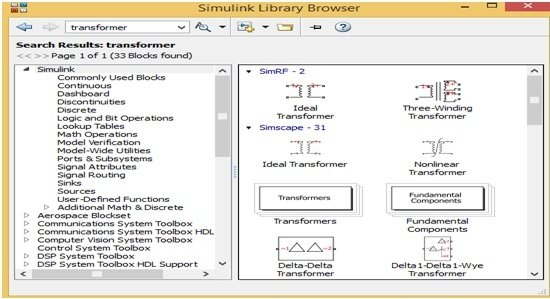Figure 1 MATLAB Simulink Library Browser

This MATLAB Simulink library browser have different components block as well as this also have different options on their Manu bar. For making a new circuit the user must have to make a new Simulink model and for this click on new model option then a new blank page would be opened shown in figure 2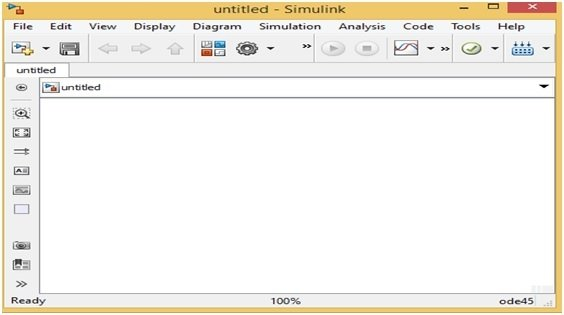Figure 2 MATLAB Simulink New Model

On this MATLAB Simulink new model, the user can make a new circuit or system. For making a new circuit the user first will chose the power electronic components like thyristor or IGBTs and for choosing this power electronics components just write the power electronics on search Manu bar then a new page would be opened shown in figure 3.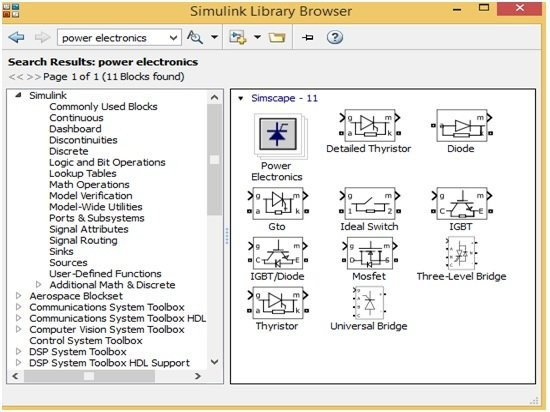Figure 3 Power Electronics Components Blocks

This new page has different power electronics components blocks shown in figure 3, now these block could be easily drag on a new Simulink model. Similarly, the user can choose the PID controller by just writing the PID on search Manu bar when he writes PI on search Manu bar then a new page again would be opened shown in figure 4,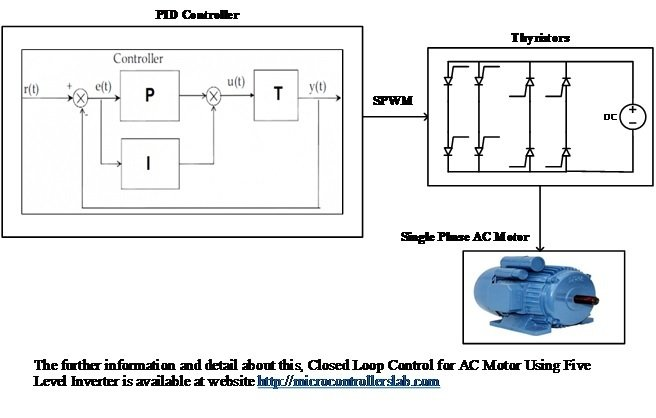Figure 4 PID Controller Blocks

Now the user can choose any one of them and then tune this according to their requirements. The user will now make a new circuit for making this closed loop control for ac motor using five level inverter according to below block diagram.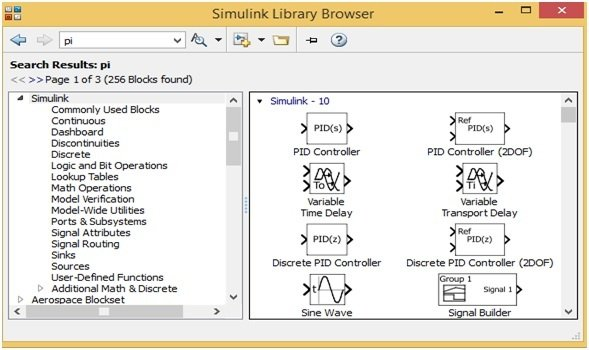Figure 5 Block Diagram of Closed Loop Control for AC Motor Using Five Level Inverter

### Simulink simulation circuit diagram of Closed Loop Control for AC Motor Using Five Level Inverter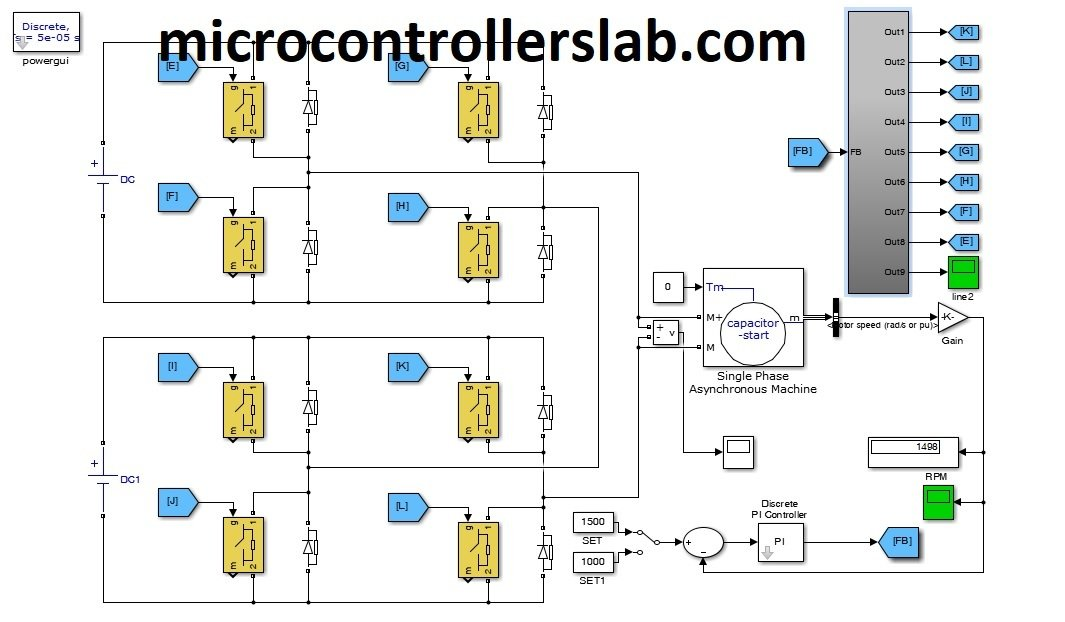### Simulation results in MATLAB simulink### Motor speed characteristics in Simulink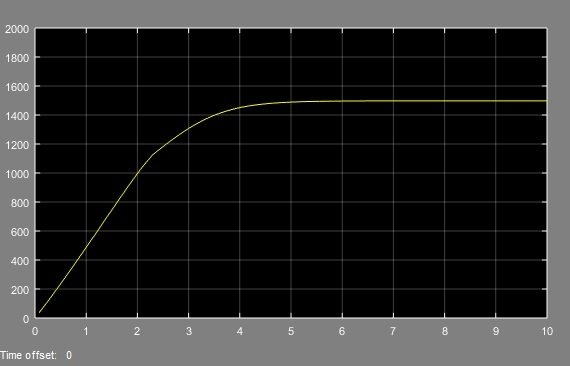Ac and dc motor components blocks are also available in MATLAB Simulink library browser and for searching them just write the motor word on search Manu bar then different motor blocks would be opened select any one like single phase ac motor block. The user can easily make this closed loop control circuit for single phase ac motor control after seeing this block diagram and run this circuit after understanding the working of this closed loop control system for ac motor using five level inverter. The five level inverter inverts the dc input into five level ac output and this ac output is produced by switching the thyristors. Thyristors are switched on /off by triggering pulse which is generated by PID controllers. SPWM (simulated pulse width modulation) technique have been used for generating the triggering pulse. In SPWM technique one sine wave and one triangular wave is compared with each through PID controller. After comparing these waves, the PID controller generates the triggering pulse for switching on or off the five level inverters thyristors. This is the simple working of this closed loop control system for ac motor control using five level inverter.

### 1 thought on “Closed Loop Control for AC Motor Using Five Level Inverter”

1. sir, i have done 3 month training in embedded system .how can i get job in company.
please suggest me . and what kind of project should be done on AVR and ARM..